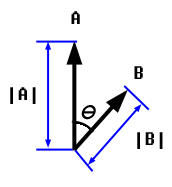# Rotating an object with mouse *math*

Ok, so basically i want my character to rotate his arm and point it where the mouse is, just like teeworlds. So i thought i could use this:

`````` A · B = |A| * |B| * cos(radians)
``````

Like this:But the thing is i’m getting wierd results. It seem to work a bit on the right side of the character but not quite…

My code to get the degree is hits:

``````
V1 = mouseX - x_pos; //V1 is the first vector
V1 = mouseY - y_pos; //x_pos is char X position
//y_pos is char Y position
V2 = 255 + x_pos;    //V2 is the second vector
V2 = 0;

degreeUp = (V1*V2); // The upper part of the formula
degreeDown = sqrt(pow(V1,2)+pow(V1,2))*sqrt(pow(V2,2)+pow(V2,2)); //crazy math, down part

handDegree = acos (degreeUp / degreeDown);	//get radians by taking acos
handDegree = (handDegree * 360) / 2 * PI;	//convert to degrees
``````

What are the weird results? It seems to work but not quite? What do you mean by that? Does it look right and then at certain angles flip to the other side or something?

You might want to look up a function called atan2. Supply a (y,x) and it will give you the degree. Something like

atan2(mousyY - y_pos, mouseX - x_pos) // check the docs, but most implementations require you to send (y,x) and not (x,y).

Also make sure you don’t need to flip your y-coordinate if your GUI framework is giving it to you where the y-axis is pointing down.

I tried atan2(mousyY - y_pos, mouseX - x_pos) and it gives me the same results.

I don’t know how to explain it.
It seems that when i just increase the mouse at the x-axis the degree increases and when i decrease at just the x-axis the degree decreases. Same for the y-axis.

Check out this it might be easier to understand:

http://leoparden.syd.kth.se/~fmi08afo/p1.avi

By debugging i noticed that mouseX and mouseY (which i capture with SDL) is fine. But i noticed that the position of x_pos and y_pos, which is position of the character, is wierd. While holding the mouse exactly on the character the mouse-coords and char coords should be almost the same right? But the x_pos is like mouseX-450 and mouseY-330, when they are on the same place… wierd? :S
I mean my stage is 640x480 and mouseX and mouseY gets coords after that, don’t know where openGL gets the wierd coords from.

** SOLVED IT ** Thanks for the help :).
The problem was i didn’t do glLoadIdentity() so i got wierd coords and i didnt convert to degrees the right way !